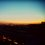# College Calc - Problem Set

$\Huge \textrm{Part I.} \\ \LARGE \textrm{Monovariable }\textrm{Monovalued }\textrm{Calculus}$

$\huge\textrm{Chapter 1. }\Large\textrm{Sequence }\textrm{and }\textrm{Function}$

$\hspace*{4ex}\LARGE \textrm{Section 1. }\large \textrm{The Reals}$

$\hspace*{8ex}$ $\textrm{Problem 01}$ ★☆☆☆☆ $\hspace*{8ex}$ $\textrm{Problem 02}$ ★★★☆☆ $\hspace*{8ex}$ $\textrm{Problem 03}$ ★★★★☆

$\hspace*{4ex}\LARGE \textrm{Section 2. }\large \textrm{Limits of Sequences}$

$\hspace*{8ex}$ $\textrm{Problem 01}$ ☆☆☆☆☆ $\hspace*{8ex}$ $\textrm{Problem 02}$ ☆☆☆☆☆ $\hspace*{8ex}$ $\textrm{Problem 03}$ ★☆☆☆☆

$\hspace*{8ex}$ $\textrm{Problem 04}$ ☆☆☆☆☆ $\hspace*{8ex}$ $\textrm{Problem 05}$ ★☆☆☆☆ $\hspace*{8ex}$ $\textrm{Problem 06}$ ★★☆☆☆

$\hspace*{8ex}$ $\textrm{Problem 07}$ ★★☆☆☆ $\hspace*{8ex}$ $\textrm{Problem 08}$ ★★★☆☆ $\hspace*{8ex}$ $\textrm{Problem 09}$ ★★★★☆

$\hspace*{8ex}$ $\textrm{Problem 10}$ ★★★☆☆ $\hspace*{8ex}$ $\textrm{Problem 11}$ ★★★☆☆ $\hspace*{8ex}$ $\textrm{Problem 12}$ ★★☆☆☆

$\hspace*{8ex}$ $\textrm{Problem 13}$ ★★☆☆☆

$\hspace*{4ex}\LARGE \textrm{Section 3. }\large \textrm{Limits of Functions}$

$\hspace*{8ex}$ $\textrm{Problem 01}$ ☆☆☆☆☆ $\hspace*{8ex}$ $\textrm{Problem 02}$ ★☆☆☆☆ $\hspace*{8ex}$ $\textrm{Problem 03}$ ★★☆☆☆

$\hspace*{8ex}$ $\textrm{Problem 04}$ ★★☆☆☆

To be continued ...Note by Boi (보이)
7 months, 3 weeks ago

This discussion board is a place to discuss our Daily Challenges and the math and science related to those challenges. Explanations are more than just a solution — they should explain the steps and thinking strategies that you used to obtain the solution. Comments should further the discussion of math and science.

When posting on Brilliant:

• Use the emojis to react to an explanation, whether you're congratulating a job well done , or just really confused .
• Ask specific questions about the challenge or the steps in somebody's explanation. Well-posed questions can add a lot to the discussion, but posting "I don't understand!" doesn't help anyone.
• Try to contribute something new to the discussion, whether it is an extension, generalization or other idea related to the challenge.
• Stay on topic — we're all here to learn more about math and science, not to hear about your favorite get-rich-quick scheme or current world events.

MarkdownAppears as
*italics* or _italics_ italics
**bold** or __bold__ bold
- bulleted- list
• bulleted
• list
1. numbered2. list
1. numbered
2. list
Note: you must add a full line of space before and after lists for them to show up correctly
paragraph 1paragraph 2

paragraph 1

paragraph 2

[example link](https://brilliant.org)example link
> This is a quote
This is a quote
    # I indented these lines
# 4 spaces, and now they show
# up as a code block.

print "hello world"
# I indented these lines
# 4 spaces, and now they show
# up as a code block.

print "hello world"
MathAppears as
Remember to wrap math in $$ ... $$ or $ ... $ to ensure proper formatting.
2 \times 3 $2 \times 3$
2^{34} $2^{34}$
a_{i-1} $a_{i-1}$
\frac{2}{3} $\frac{2}{3}$
\sqrt{2} $\sqrt{2}$
\sum_{i=1}^3 $\sum_{i=1}^3$
\sin \theta $\sin \theta$
\boxed{123} $\boxed{123}$

## Comments

Sort by:

Top Newest

Awesome! Hope you keep updating it :)

- 6 months ago

Log in to reply

Thanks :) I plan to !

- 5 months, 3 weeks ago

Log in to reply

×

Problem Loading...

Note Loading...

Set Loading...